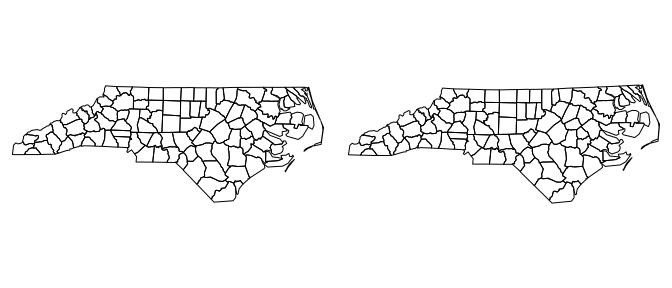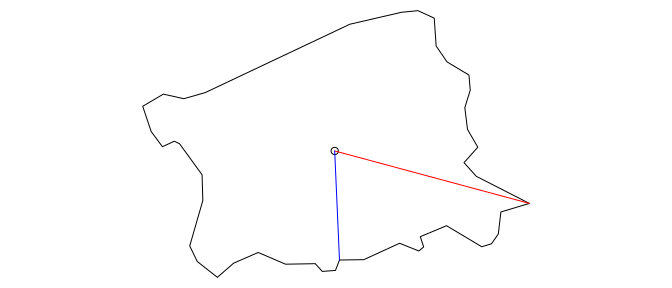Spatial Utility Functions

2021-06-19

This package contains convenience functions for carrying out GIS operations that I have repeatedly encountered in my research. The following packages are used in this vignette:

library(sf)
library(raster)
library(RWmisc)

Weighting raster values by overlapping polygons

The overlap.weight function allows you to weight the values of a raster cell by the inverse of the number of polygons that overlap the cell. This is useful when, e.g., calculating the population of ethnic group settlement areas when different group settlement areas can overlap one another. The count argument allows the count of overlapping polygons to be returned instead of the weighted cell values. Note that this converts any cells not covered by at least one polygon to NA.

## create three overlapping squares
polys_t <- st_sfc(list(st_polygon(list(rbind(c(2,2), c(2,6), c(6,6),
c(6,2), c(2, 2)))),
st_polygon(list(rbind(c(8,8), c(4,8), c(4,4),
c(8,4), c(8,8)))),
st_polygon(list(rbind(c(3,3), c(3,7), c(7,7),
c(7,3), c(3,3))))),
crs = st_crs('OGC:CRS84'))

## create raster
raster_t <- raster(nrows = 6, ncols = 6, xmn = 2, xmx = 8, ymn = 2, ymx = 8,
plot(projectUTM(nc)$geometry)Maximum and minimum distance from point(s) to a polygon The point.poly.dist function computes the maximum or minimum distance from a point or set of points to a polygon. It correctly calculates distances for both geographic and projected data. ## create north carolina centroids nc_centroids <- st_centroid(nc) #> Warning in st_centroid.sf(nc): st_centroid assumes attributes are constant over #> geometries of x ## calculate maximum distance point.poly.dist(nc_centroids[53,]$geometry, nc[53,]$geometry, max = TRUE) #> Loading required namespace: geosphere #> 33565.04 [m] The following illustration depicts the line connecting the centroid of the polygon to the farthest point on the polygon (red) and the nearest point on the polygon (blue). nc_points <- st_geometry(nc[53,]) %>% st_cast('POINT') farthest_ind <- st_distance(nc_points, nc_centroids[53,]) %>% which.max() farthest_point <- rbind(st_coordinates(nc_points[farthest_ind]), st_coordinates(nc_centroids[53,])) %>% st_linestring() nearest_ind <- st_distance(nc_points, nc_centroids[53,]) %>% which.min() nearest_point <- rbind(st_coordinates(nc_points[nearest_ind]), st_coordinates(nc_centroids[53,])) %>% st_linestring() ## plot par(mar = rep(0,4)) plot(nc[53,]$geometry)
plot(nc_centroids[53,]$geometry, add = TRUE) plot(farthest_point, add = TRUE, col = 'red') plot(nearest_point, add = TRUE, col = 'blue')Carrying out the same calculations using built-in sf functions takes roughly twice as long to execute. microbenchmark::microbenchmark(pk = point.poly.dist(nc_centroids[53,]$geometry,
nc[53,]\$geometry, max = TRUE),
sf = st_distance(st_cast(st_geometry(nc[53,]),
'POINT')[farthest_ind],
nc_centroids[53,]),
times = 100)
#> Unit: milliseconds
#>  expr      min       lq     mean   median       uq      max neval
#>    pk 4.725473 4.750896 4.976703 4.807256 4.922799 8.048516   100
#>    sf 5.532677 5.576869 5.822413 5.605588 5.652372 9.909867   100